### Compound Interest Tricks, Tips & Results-1

In the first two articles for Compound Interest, we have covered the important concepts for this topic. In this article, we shift our focus from concepts to Compound Interest tricks. The focus of this article is purely to explore different compound interest tricks, shortcuts and results that can be used to solve problems quickly. There are quite a few examinations (especially exams that feature objective type questions) which require quick problem solving skills and need you to be on top of your game. It is in these exams where you need to solve questions in the quickest possible time that you need need to employ these compound interest tricks.

Compound Interest Tricks: Results related to Multiple Compounding in a year:

In each of the following results, we use the following denotations:
A = future value
P = principal amount (initial investment)
r = annual nominal interest rate
n = number of times the interest is compounded per year
t = number of years for which the money is borrowed

Compound Interest Trick 1: If interest is not compounded yearly then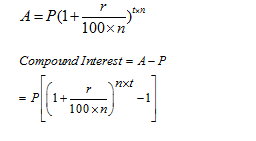where

n= number of times compounding is done
if compounding is done half yearly then n = 2
if compounding is done quarterly  then n = 4
when compounded monthly then n=12

Amount for Half Yearly Compounding, A = P {1+(R/2)/ 100}2T
(compound interest applied two times in a year)
Like Half Yearly Compound Interest, we can calculate the amount for Quarterly Compounding.
Amount for Quarterly Compounding,A = P {1+(R/4)/ 100}4T

Example 1: Sona deposited Rs. 4000 in a bank for 2 years at 5% p.a.rate. Find the amount received by her from the bank if interest is compounded half yearly.

Solution:
Principal value = Rs. 4000
Rate = 5%
Time = 2 years
Since the interest is compounded half yearly so 2 years = 4 times in two years
So, we have
A = P {1+(R/2)/ 100}2T
A= 4000{1+ (5/2)/100}4
A = 4000 x 41/40 x 41/40 x 41/40 x 41/40
A = Rs. 4415.25
So, Sona received Rs. 4415.25 from the bank after two years

Example 2: Manpreet lent Rs 5000 to Richa at 10% rate for 1 year. But she told her that she will take her money on compound interest. Find the amount of interest received by Manpreet when compounded quarterly.

Solution:
Principal value = Rs. 5000
Rate = 10%
Time = 1 year
The interest is compounded quarterly, that is 4 times in 1 year.
We use the formula=A = P {1+(R/4)/ 100}4T
A= 5000{1+ (10/4)/100}4
A = 5000 x 41/40 x 41/40 x 41/40 x 41/40
A = Rs. 5519.064
So, Manpreet received Rs. 5519.064 from Richa and the total amount of interest received by her is 5519.064 – 5000 = Rs. 519.06

Compound Interest Tricks: When the rate % is not same for every year and interest is taken on yearly basis then amount can be calculated as: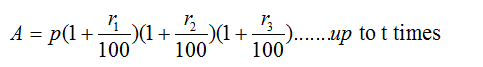Example 3:  Find the compound interest on 16000 in 2 years , while rate of interest at first year is 3% and for the second year is 4%.

Solution: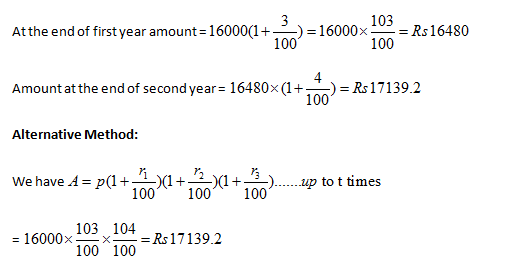Compound Interest Tricks: When interest is compounded yearly and time is given in fraction

If time is given in mixed fraction

Use formula,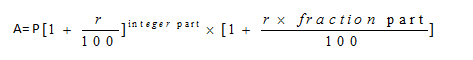Example 4: The compound interest on Rs. 8,000 at 15% per annum for 2 years 4 months, compounded annually is:

Solution: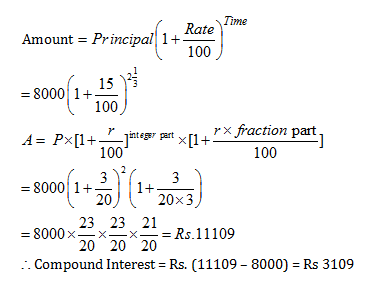### Compound Interest Tricks: Practice Questions

Question 1: A certain sum, invested at 4% per annum compound interest, compounded half – yearly, amounts to Rs 7,803 at the end of one year. The sum is

A. Rs 7,000
B. Rs 7,200
C. Rs 7,500
D. Rs 7,700

Option C

Let the sum be Rs. P.

As, the interest is compounded half-yearly.

R = 2%, T = 2 half years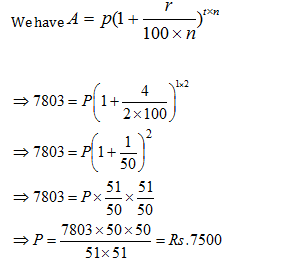Question 2: If the rate of interest be 4% per annum for first year, 5% per an­num for second year and 6% per annum for third year, then the compound interest of Rs. 10,000 for 3 years will be

A. Rs. 1,600
B. Rs. 1,625.80
C. Rs. 1,575.20
D. Rs. 2,000

Option C

When the rate of interest is different for the different years, we can use the following formula to find the amount.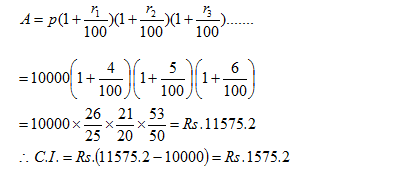Question 3: The compound interest on Rs. 16,000 for 9 months at 20% per annum, interest being com­pounded quarterly, is

A. Rs. 2,520
B. Rs. 2,524
C. Rs. 2,522
D. Rs. 2,518

Option C

The interest is compounded quarterly. So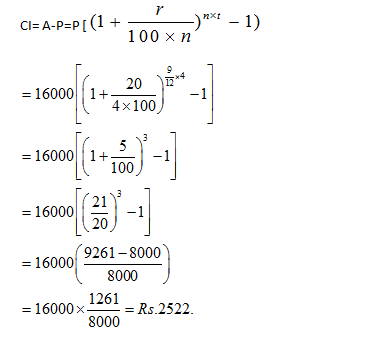Question 4: The compound interest on Rs. 6,000 at 10% per annum for 3/2 years, when the interest be¬ing compounded annually, is
A. Rs. 930
B. Rs. 870
C. Rs. 910
D. Rs. 900

Option A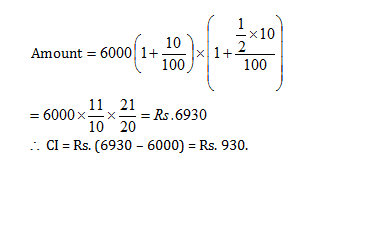#### Compound Interest Practice Tests

Get Posts Like This Sent to your Email
Updates for Free Live sessions and offers are sent on mail. Don't worry: we do not send too many emails..:)
Get Posts Like This Sent to your Email
Updates for Free Live sessions and offers are sent on mail. Don't worry: we do not send too many emails..:)